faster rcnn中损失函数（二）—— Smoooh L1 Loss的讲解

1. 使用Smoooh L1 Loss的原因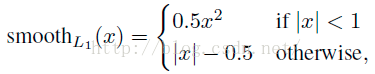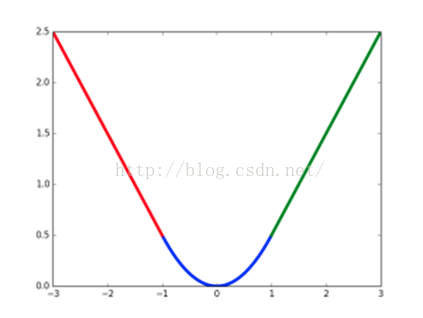2.

SmoothL1LossLayer 计算一张图片的损失函数，对应于下图的加号右边部分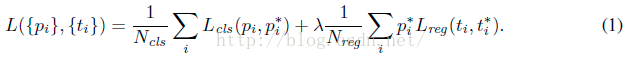i是mini-batch的anchor的索引。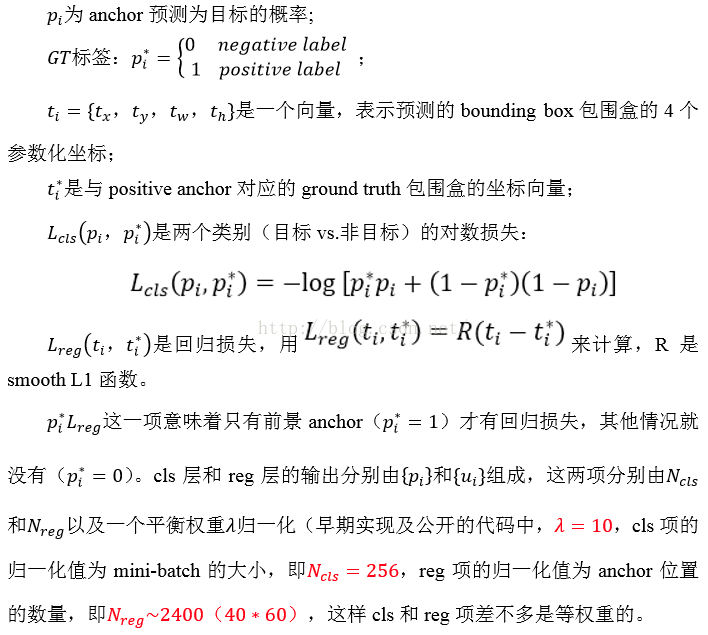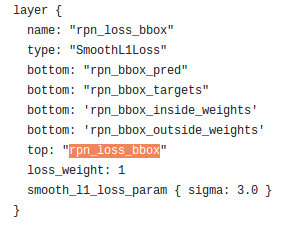ti是一个向量，预测坐标 = (tx,ty,tw,th)

ti*是一个向量，是gt包围盒的坐标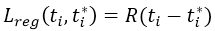R表示的下一个图那样的分段函数。

bottom预测坐标，对应于下图的ti，即rpn_bbox_pred层返回的预测的与初始anchor的偏移。

bottomtarget坐标，对应于下图的ti*，即anchor_target_layer类型的rpn_data层返回的，rpn_bbox_target，gt与初始每个anchor的偏移。

bottominside，有物体(fg)时为1，否则为0，对应于上图的pi*

bottomoutside，没有前景（fg）也没有后景（bg）的为0，其他为1/（bg+fg）=Ncls对应于加号右边的系数部分（但其实这个地方我本人还是不懂，因为论文上说的系数都是一些固定的值，如入=10。初始代码一直在更新，估计又换了别的方法。不论如何，在现在的代码中outside是乘以了后面的结果）

Lreg的公式就是下图，另 x=ti - ti*，

other:   |x|-0.5/(sigma**2)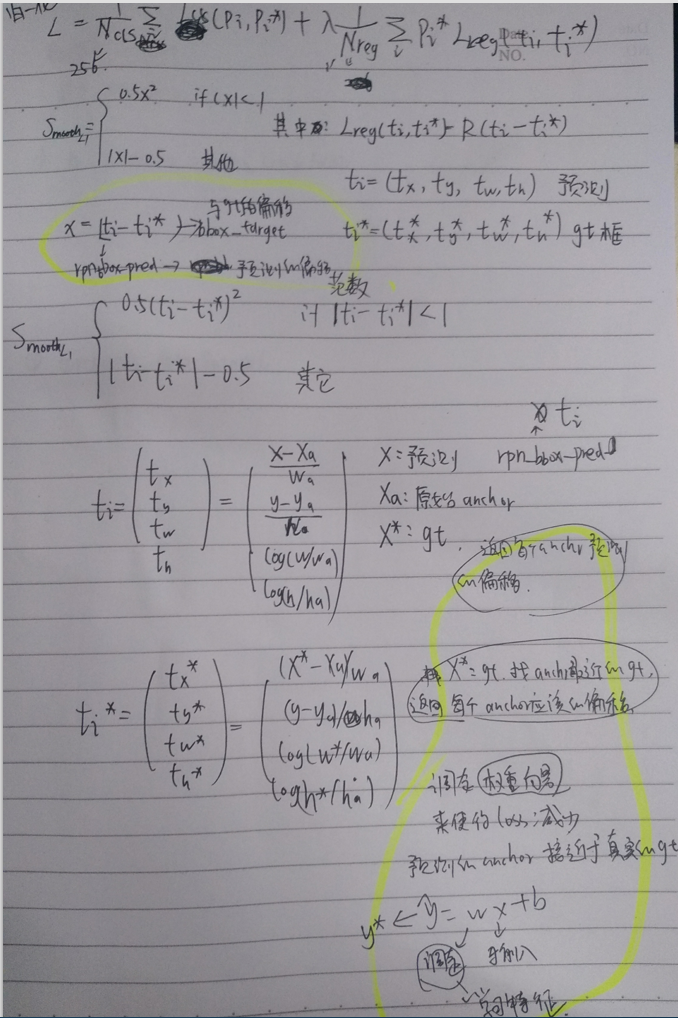def _smooth_l1_loss(bbox_pred, bbox_targets, bbox_inside_weights, bbox_outside_weights, sigma=1.0, dim=):

sigma_2 = sigma ** 2
box_diff = bbox_pred - bbox_targets### B,9*4,H,W      B,9*4,H,W都是variable
in_box_diff = bbox_inside_weights * box_diff# 内部正样本的diff
abs_in_box_diff = torch.abs(in_box_diff)
#detach返回的Variable和原始的Variable公用同一个data tensor。
smoothL1_sign = (abs_in_box_diff < 1. / sigma_2).detach().float()

in_loss_box = torch.pow(in_box_diff, 2) * (sigma_2 / 2.) * smoothL1_sign \
+ (abs_in_box_diff - (0.5 / sigma_2)) * (1. - smoothL1_sign)
out_loss_box = bbox_outside_weights * in_loss_box#bbox_outside_weights 正负样本都是1/256 背景为0
#注意学习loss的相加，按照轴
loss_box = out_loss_box
for i in sorted(dim, reverse=True):
loss_box = loss_box.sum(i)
loss_box = loss_box.mean()
return loss_box

detach能用来干啥

, 两个关系是这样的 y=A(x),z=B(y) 现在我们想用 z.backward() 来为 B 网络的参数来求梯度，但是又不想求 A

# y=A(x), z=B(y) 求B中参数的梯度，不求A中参数的梯度
# 第一种方法
y = A(x)
z = B(y.detach())
z.backward()

# 第二种方法
y = A(x)
y.detach_()
z = B(y)
z.backward()